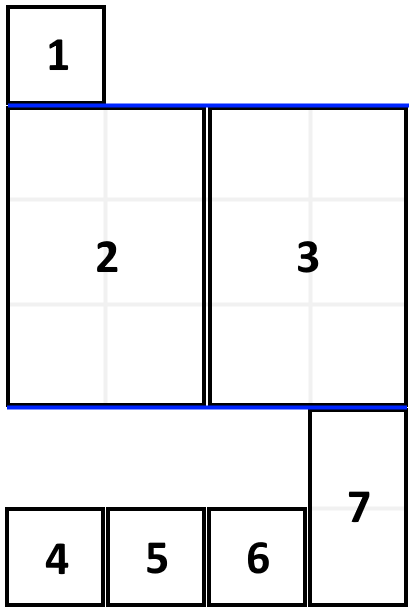LeetCode: 1105. 填充书架¶

1、题目描述¶3 层书架的高度和为 1 + 3 + 2 = 6 。

• 1 <= books.length <= 1000
• 1 <= books[i] <= shelf_width <= 1000
• 1 <= books[i] <= 1000

2、解题思路¶

• 动态规划

dp[i]表示 books[i:] 最小的高度

while index >= back and current_thickness <= shelf_width:
dp[index + 1] = min(dp[index + 1], dp[index - back] + current_height)
back += 1
current_thickness += books[index - back]
current_height = max(current_height, books[index - back])

class Solution:
def minHeightShelves(self, books: List[List[int]], shelf_width: int) -> int:
inf = float("inf")

dp = [inf] * (len(books) + 1)
dp = 0
books.reverse()
for index, (thickness, height) in enumerate(books):
current_thickness = thickness
current_height = height
back = 0
while index >= back and current_thickness <= shelf_width:
dp[index + 1] = min(dp[index + 1], dp[index - back] + current_height)
back += 1
current_thickness += books[index - back]
current_height = max(current_height, books[index - back])
return dp[-1]